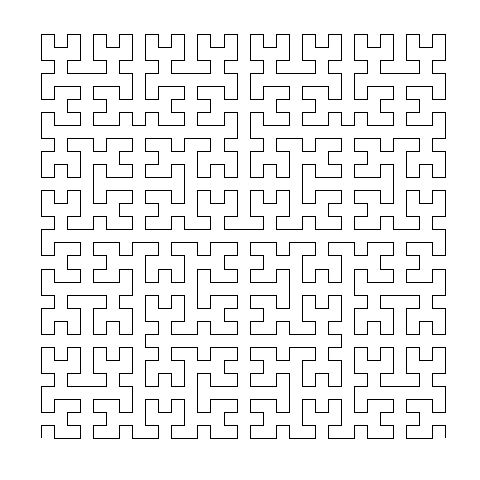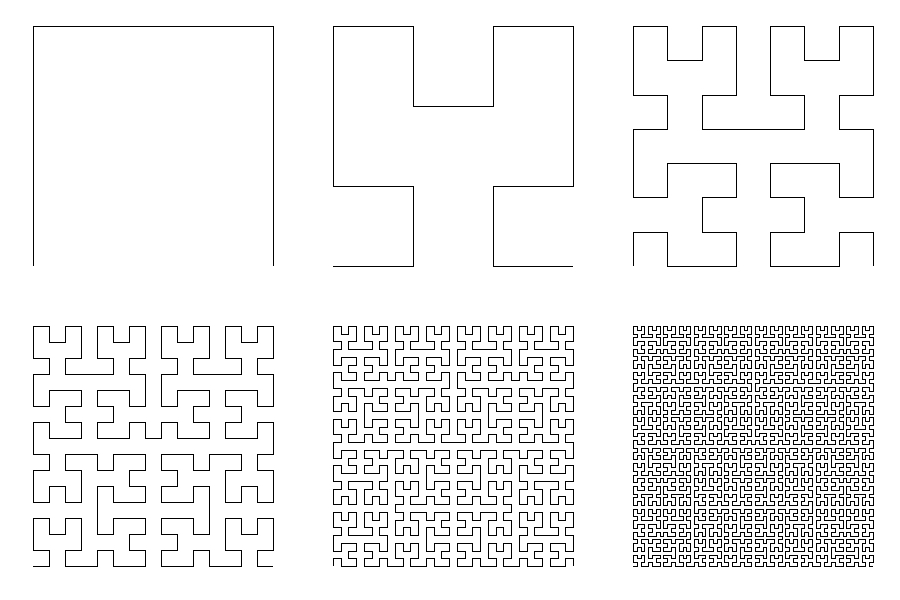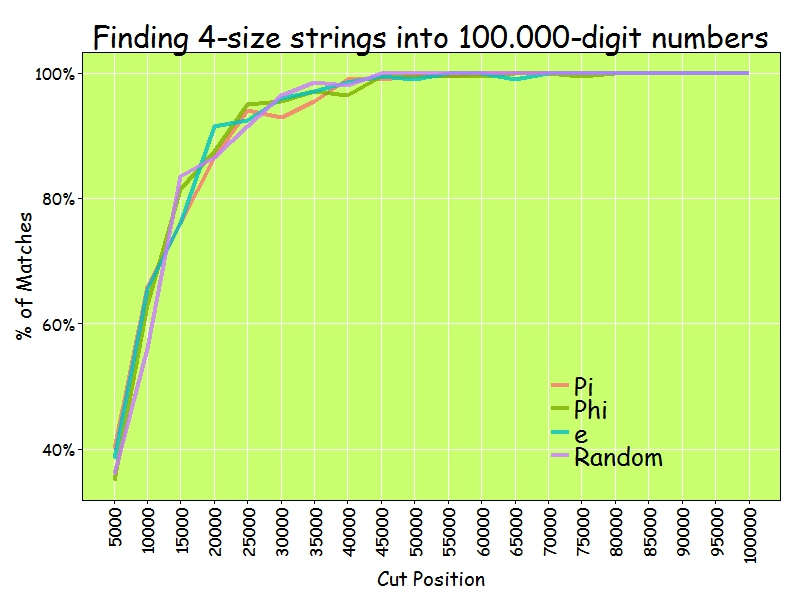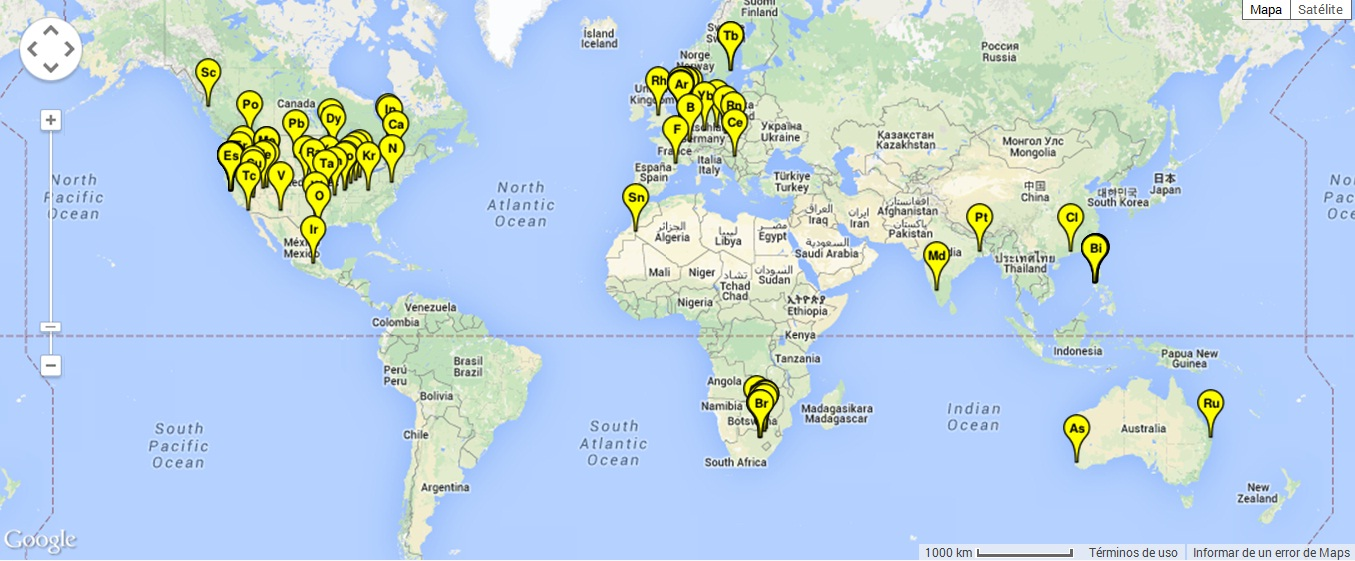The Meeting Point Locator

Hi Hillary, It’s Donald, would you like to have a beer with me in La Cabra Brewing, in Berwyn, Pensilvania? (Hypothetical utilization of The Meeting Point Locator)

Finding a place to have a drink with someone may become a difficult task. It is quite common that one of them does not want to move to the other’s territory. I am sure you have faced to this situation many times. With The Meeting Point Locator this will be no longer an issue, since it will give you a list of equidistant bars and coffees to any two given locations. Let’s see an example.

I do not know if Hillary Clinton and Donald Trump have met each other after the recent elections in United States, but the will probably do. Let’s suppose Hillary doesn’t want to go to The White House and that Donald prefers another place instead Hillary’s home. No problem at all. According to this, Hillary lives in Chappaqua, New York and Donald will live in The White House, Washington (although he supposedly won’t do full time as he announced recently). These two locations are the only input that The Meeting Point Locator needs to purpose equidistant places where having a drink. This is how it works:

• Generates a number of coordinates on the great circle which passes through the midpoint of the original locations and is orthogonal to the rhumb defined by them; the number of points depends on the distance between the original locations.
• Arranges these coordinates according to the distance to the original locations, from the nearest to the most distant.
• Depending also on the distance of the original locations, defines a radius to search around each point generated on the great circle (once calculated, this radius is constant for all searches).
• Starting from the nearest point, looks for a number of places (20 by default) to have a drink using the radius calculated previously. To do this, it calls to the Google Places API. Once the number of locations is reached, the proccess stops.

This map shows the places purposed for Hillary and Donald (blue points) as well as the original locations (red ones). You can make zoom in for details:

These are the 20 closest places to both of them:Some other examples of the utility of The Meeting Point Locator:

• Pau Gasol (who lives in San Antonio, Texas) and Marc Gasol (in Memphis, Tennessee) can meet in The Draft Sports Bar, in Leesville (Louisiana) to have a beer while watching a NBA match. It is 537 kilometers far from both of them.
• Bob Dylan (who lives in Malibu, California) and The Swedish Academy (placed in Stockholm, Sweden) can smooth things over drinking a caipirinha in Bar São João, in Tremedal (Brasil)only 9.810 kilometers far from both of them.
• Spiderman (placed in New York City) and Doraemon (in Tokio, Japan) can meet in Andreyevskaya, in Stroitel (Russia) to have a have a hot drink. Since they are superheroes, they will cover the 9.810 kilometers of separation in no time at all.

I faced with two challenges to do this experiment: how to generate the orthogonal great circle from two given locations and how to define radius and number of points over this circle to do searchings. I will try to explain in depth both things in the future in another post.

You will find the code below. To make it work, do not forget to get your own key for Google Places API Web Service here. I hope this tool will be helpful for someone; if yes, do not hesitate to tell it to me.

library(httr)
library(jsonlite)
library(dplyr)
library(ggmap)
library(geosphere)
library(DT)
library(leaflet)

# Write both addresses here (input)
place1="Chappaqua, New York, United States of America"
place2="The White House, Washington DC, United States of America"

# Call to Google Maps API to obtain coordinates of previous addresses
p1=geocode(place1, output = "latlon")
p2=geocode(place2, output = "latlon")

# To do searchings I need a radius
ifelse(distGeo(p1, p2)>100000, 2500, 1000))

# And a number of points
npoints=ifelse(distGeo(p1, p2)>1000000, 2002,
ifelse(distGeo(p1, p2)>100000, 7991, 19744))

# Place here the Google Places API Key
key="PLACE_YOUR_OWN_KEY_HERE"

# Build the url to look for bars and cafes with the previous key
url2="&types=cafe|bar&key="

# This is to obtain the great circle orthogonal to the rhumb defined by input locations
# and which passes over the midpoint. I will explain this step in the future
mid=midPoint(p1, p2)
dist=distGeo(p1, p2)
x=p1
y=p2
while(dist>1000000)
{
x=midPoint(mid, x)
y=midPoint(mid, y)
dist=distGeo(x, y)
}

bea=bearingRhumb(x, y)
points=greatCircle(destPoint(p=mid, b=bea+90, d=1), mid, n=npoints)

# Arrange the points dependning on the distance to the input locations
data.frame(dist2p1=apply(points, 1, function (x) distGeo(p1, x)),
dist2p2=apply(points, 1, function (x) distGeo(p2, x))) %>%
mutate(order=apply(., 1, function(x) {max(x)})) %>%
cbind(points) %>%
arrange(order) -> points

# Start searchings
nlocs=0 # locations counter (by default stops when 20 is reached)
niter=1 # iterations counter (if greater than number of points on the great circle, stops)
results=data.frame()
while(!(nlocs>=20 | niter>npoints)) {
print(niter)
url %>%
gsub("lat", points[niter, 'lat'], .) %>%
gsub("lon", points[niter, 'lon'], .) %>%
GET %>%
content("text") %>%
fromJSON -> retrieve

df=data.frame(lat=retrieve\$results\$geometry\$location\$lat,
lng=retrieve\$results\$geometry\$location\$lng,
name=retrieve\$results\$name,
results %>% rbind(df)->results

nlocs=nlocs+nrow(df)
niter=niter+1
}

# I prepare results to do a Data Table
data.frame(dist2p1=apply(results, 1, function (x) round(distGeo(p1, c(as.numeric(x), as.numeric(x)))/1000, digits=1)),
dist2p2=apply(results, 1, function (x) round(distGeo(p2, c(as.numeric(x), as.numeric(x)))/1000, digits=1))) %>%
mutate(mx=apply(., 1, function(x) {max(x)})) %>%
cbind(results) %>%
arrange(mx) %>%
mutate(rank=row_number()) %>%
select(-mx)-> resultsDT

# This is the Data table
datatable(resultsDT,
class = 'cell-border stripe',
rownames = FALSE,
options = list(pageLength = 5),
colnames = c('Distance to A (Km)',
'Distance to B (Km)',
'Latitude',
'Longitude',
'Name',
'Rank'))

# Map with the locations using leaflet
resultsDT %>%
leaflet() %>%
lng=resultsDT\$lng, lat=resultsDT\$lat,
color = "blue",
stroke = FALSE, fillOpacity = 0.5,
")
) %>%
lng=p1\$lon, lat=p1\$lat,
color = "red",
stroke = FALSE, fillOpacity = 0.5,
popup=paste("<b>Place 1</b>", place1, sep="
")
)%>%
lng=p2\$lon, lat=p2\$lat,
color = "red",
stroke = FALSE, fillOpacity = 0.5,
popup=paste("<b>Place 2</b>", place2, sep="
")
)

Gummy Worms

Just keep swimming (Dory in Finding Nemo)

Inspired by this post, I decided to create gummy worms like this:Or these:

When I was young I used to eat them.

Do you want to try? This is the code:

library(rgl)
library(RColorBrewer)
t=seq(1, 6, by=.04)
f = function(a, b, c, d, e, f, t) exp(-a*t)*sin(t*b+c)+exp(-d*t)*sin(t*e+f)
v1=runif(6,0,1e-02)
v2=runif(6, 2, 3)
v3=runif(6,-pi/2,pi/2)
open3d()
spheres3d(x=f(v1, v2, v3, v1, v2, v3, t),
y=f(v1, v2, v3, v1, v2, v3, t),
z=f(v1, v2, v3, v1, v2, v3, t),

The Hype Bubble Map for Dog Breeds

In the whole history of the world there is but one thing that money can not buy… to wit the wag of a dog’s tail (Josh Billings)

In this post I combine several things:

• Simple webscraping to read the list of companion dogs from Wikipedia. I love rvest package to do these things
• Google Trends queries to download the evolution of searchings of breeds during last 6 months. I use gtrendsR package to do this and works quite well
• A dinamic Highchart visualization using the awesome highcharter package

The experiment is based on a simple idea: what people search on the Internet is what people do. Can be Google Trends an useful tool to know which breed will become fashionable in the future? To be honest, I don’t really know but I will make my own bet.

What I have done is to extract last 6 months of Google trends of this list of companion breeds. After some simple text mining, I divide the set of names into 5-elements subsets because Google API doesn’t allow searchings with more than 5 items. The result of the query to Google trends is a normalized time series, meaning the 0 – 100 values are relative, not absolute, measures. This is done by taking all of the interest data for your keywords and dividing it by the highest point of interest for that date range. To make all 5-items of results comparable I always include King Charles Spaniel breed in all searchings (as a kind of undercover agent I will use to compare searching levels). The resulting number is my “Level” Y-Axis of the plot. I limit searchings to code=”0-66″ which is restrict results to Animals and pets category. Thanks, Philippe, for your help in this point. I also restrict rearchings To the United States of America.

There are several ways to obtain an aggregated trend indicator of a time series. My choice here was doing a short moving average order=2 to the resulting interest over time obtained from Google. The I divide the weekly variations by the smoothed time series. The trend indicator is the mean of these values. To obtains a robust indicator, I remove outliers of the original time series. This is my X-axis.

I discovered recently a wonderful package called highcharter which allows you to create incredibly cool dynamic visualizations. I love it and I could not resist to use it to do the previous plot with the look and feel of The Economist:

Inspired by Gartner’s Hype Cycle of Emerging Technologies I distinguish two sets of dog breeds:

• Plateau of Productivity Breeds (succesful breeds with very high level indicator and possitive trend): Golden Retriever, Pomeranian, Chihuahua, Collie and Shih Tzu.
• Innovation Trigger Breeds (promising dog breeds with very high trend indicator and low level): Mexican Hairless Dog, Keeshond, West Highland White Terrier and German Spitz.

And here comes my prediction. After analyzing the set Innovation Trigger Breeds, my bet is Keeshond will increase its popularity in the nearly future: don’t you think it is lovely?Photo by Terri BrownFlickr: IMG_4723, CC BY 2.0

Here you have the code:

library(gtrendsR)
library(rvest)
library(dplyr)
library(stringr)
library(forecast)
library(outliers)
library(highcharter)
library(ggplot2)
library(scales)

x="https://en.wikipedia.org/wiki/Companion_dog"
html_nodes("ul:nth-child(19)") %>%
html_text() %>%
strsplit(., "\n") %>%
unlist() -> breeds
breeds=iconv(breeds[breeds!= ""], "UTF-8")
gconnect(usr, psw)
#Reference
ref="King Charles Spaniel"
#New set
breeds=setdiff(breeds, ref)
#Subsets. Do not worry about warning message
sub.breeds=split(breeds, 1:ceiling(length(breeds)/4))
results=list()
for (i in 1:length(sub.breeds))
{
res <- gtrends(unlist(union(ref, sub.breeds[i])),
start_date = Sys.Date()-180,
cat="0-66",
geo="US")
results[[i]]=res
}
trends=data.frame(name=character(0), level=numeric(0), trend=numeric(0))
for (i in 1:length(results))
{
df=results[[i]]\$trend
lr=mean(results[[i]]\$trend[,3]/results[]\$trend[,3])
for (j in 3:ncol(df))
{
s=rm.outlier(df[,j], fill = TRUE)
t=mean(diff(ma(s, order=2))/ma(s, order=2), na.rm = T)
l=mean(results[[i]]\$trend[,j]/lr)
trends=rbind(data.frame(name=colnames(df)[j], level=l, trend=t), trends)
}
}

trends %>%
group_by(name) %>%
summarize(level=mean(level), trend=mean(trend*100)) %>%
filter(level>0 & trend > -10 & level<500) %>%
na.omit() %>%
mutate(name=str_replace_all(name, ".US","")) %>%
mutate(name=str_replace_all(name ,"[[:punct:]]"," ")) %>%
rename(
x = trend,
y = level
) -> trends
trends\$y=(trends\$y/max(trends\$y))*100
#Dinamic chart as The Economist
highchart() %>%
hc_title(text = "The Hype Bubble Map for Dog Breeds") %>%
hc_subtitle(text = "According Last 6 Months of Google Searchings") %>%
hc_xAxis(title = list(text = "Trend"), labels = list(format = "{value}%")) %>%
hc_yAxis(title = list(text = "Level")) %>%
hc_add_series(data = list.parse3(trends), type = "bubble", showInLegend=FALSE, maxSize=40) %>%
hc_tooltip(formatter = JS("function(){
return ('<b>Trend: </b>' + Highcharts.numberFormat(this.x, 2)+'%' + '<br><b>Level: </b>' + Highcharts.numberFormat(this.y, 2) + '<br><b>Breed: </b>' + this.point.name)
}"))

Going Bananas With Hilbert

It seemed that everything is in ruins, and that all the basic mathematical concepts have lost their meaning (Naum Vilenkin, Russian mathematician, regarding to the discovery of Peano’s curve)

Giuseppe Peano found in 1890 a way to draw a curve in the plane that filled the entire space: just a simple line covering completely a two dimensional plane. Its discovery meant a big earthquake in the traditional structure of mathematics. Peano’s curve was the first but not the last: one of these space-filling curves was discovered by Hilbert and takes his name. It is really beautiful:Hilbert’s curve can be created iteratively. These are the first six iterations of its construction:As you will see below, R code to create Hilbert’s curve is extremely easy. It is also very easy to play with the curve, altering the order in which points are sorted. Changing the initial matrix(1) by some other number, resulting curves are quite appealing:

Let’s go futher. Changing ggplot geometry from geom_path to geom_polygon generate some crazy pseudo-tessellations:

And what if you change the matrix exponent?

And what if you apply polar coordinates?

We started with a simple line and with some small changes we have created fantastical images. And all these things only using black and white. Do you want to add some colors? Try with the following code (if you draw something interesting, please let me know):

library(reshape2)
library(dplyr)
library(ggplot2)
opt=theme(legend.position="none",
panel.background = element_rect(fill="white"),
panel.grid=element_blank(),
axis.ticks=element_blank(),
axis.title=element_blank(),
axis.text=element_blank())
hilbert = function(m,n,r) {
for (i in 1:n)
{
tmp=cbind(t(m), m+nrow(m)^2)
m=rbind(tmp, (2*nrow(m))^r-tmp[nrow(m):1,]+1)
}
melt(m) %>% plyr::rename(c("Var1" = "x", "Var2" = "y", "value"="order")) %>% arrange(order)}
# Original
ggplot(hilbert(m=matrix(1), n=1, r=2), aes(x, y)) + geom_path()+ opt
ggplot(hilbert(m=matrix(1), n=2, r=2), aes(x, y)) + geom_path()+ opt
ggplot(hilbert(m=matrix(1), n=3, r=2), aes(x, y)) + geom_path()+ opt
ggplot(hilbert(m=matrix(1), n=4, r=2), aes(x, y)) + geom_path()+ opt
ggplot(hilbert(m=matrix(1), n=5, r=2), aes(x, y)) + geom_path()+ opt
ggplot(hilbert(m=matrix(1), n=6, r=2), aes(x, y)) + geom_path()+ opt
# Changing order
ggplot(hilbert(m=matrix(.5), n=5, r=2), aes(x, y)) + geom_path()+ opt
ggplot(hilbert(m=matrix(0), n=5, r=2), aes(x, y)) + geom_path()+ opt
ggplot(hilbert(m=matrix(tan(1)), n=5, r=2), aes(x, y)) + geom_path()+ opt
ggplot(hilbert(m=matrix(3), n=5, r=2), aes(x, y)) + geom_path()+ opt
ggplot(hilbert(m=matrix(-1), n=5, r=2), aes(x, y)) + geom_path()+ opt
ggplot(hilbert(m=matrix(log(.1)), n=5, r=2), aes(x, y)) + geom_path()+ opt
ggplot(hilbert(m=matrix(-15), n=5, r=2), aes(x, y)) + geom_path()+ opt
ggplot(hilbert(m=matrix(-0.001), n=5, r=2), aes(x, y)) + geom_path()+ opt
# Polygons
ggplot(hilbert(m=matrix(log(1)), n=4, r=2), aes(x, y)) + geom_polygon()+ opt
ggplot(hilbert(m=matrix(.5), n=4, r=2), aes(x, y)) + geom_polygon()+ opt
ggplot(hilbert(m=matrix(tan(1)), n=5, r=2), aes(x, y)) + geom_polygon()+ opt
ggplot(hilbert(m=matrix(-15), n=4, r=2), aes(x, y)) + geom_polygon()+ opt
ggplot(hilbert(m=matrix(-25), n=4, r=2), aes(x, y)) + geom_polygon()+ opt
ggplot(hilbert(m=matrix(0), n=4, r=2), aes(x, y)) + geom_polygon()+ opt
ggplot(hilbert(m=matrix(1000000), n=4, r=2), aes(x, y)) + geom_polygon()+ opt
ggplot(hilbert(m=matrix(-1), n=4, r=2), aes(x, y)) + geom_polygon()+ opt
ggplot(hilbert(m=matrix(-.00001), n=4, r=2), aes(x, y)) + geom_polygon()+ opt
# Changing exponent
gplot(hilbert(m=matrix(log(1)), n=4, r=-1), aes(x, y)) + geom_polygon()+ opt
ggplot(hilbert(m=matrix(.5), n=4, r=-2), aes(x, y)) + geom_polygon()+ opt
ggplot(hilbert(m=matrix(tan(1)), n=4, r=6), aes(x, y)) + geom_polygon()+ opt
ggplot(hilbert(m=matrix(-15), n=3, r=sin(2)), aes(x, y)) + geom_polygon()+ opt
ggplot(hilbert(m=matrix(-25), n=4, r=-.0001), aes(x, y)) + geom_polygon()+ opt
ggplot(hilbert(m=matrix(0), n=4, r=200), aes(x, y)) + geom_polygon()+ opt
ggplot(hilbert(m=matrix(1000000), n=3, r=.5), aes(x, y)) + geom_polygon()+ opt
ggplot(hilbert(m=matrix(-1), n=4, r=sqrt(2)), aes(x, y)) + geom_polygon()+ opt
ggplot(hilbert(m=matrix(-.00001), n=4, r=52), aes(x, y)) + geom_polygon()+ opt
# Polar coordinates
ggplot(hilbert(m=matrix(1), n=4, r=2), aes(x, y)) + geom_polygon()+ coord_polar()+opt
ggplot(hilbert(m=matrix(-1), n=5, r=2), aes(x, y)) + geom_polygon()+ coord_polar()+opt
ggplot(hilbert(m=matrix(.1), n=2, r=.5), aes(x, y)) + geom_polygon()+ coord_polar()+opt
ggplot(hilbert(m=matrix(1000000), n=2, r=.1), aes(x, y)) + geom_polygon()+ coord_polar()+opt
ggplot(hilbert(m=matrix(.25), n=3, r=3), aes(x, y)) + geom_polygon()+ coord_polar()+opt
ggplot(hilbert(m=matrix(tan(1)), n=5, r=1), aes(x, y)) + geom_polygon()+ coord_polar()+opt
ggplot(hilbert(m=matrix(1), n=4, r=1), aes(x, y)) + geom_polygon()+ coord_polar()+opt
ggplot(hilbert(m=matrix(log(1)), n=3, r=sin(2)), aes(x, y)) + geom_polygon()+ coord_polar()+opt
ggplot(hilbert(m=matrix(-.0001), n=4, r=25), aes(x, y)) + geom_polygon()+ coord_polar()+opt

Going Bananas #2: A Needle In A Haystack

Now I’m gonna tell my momma that I’m a traveller, I’m gonna follow the sun (The Sun, Parov Stelar)

Inspired by this book I read recently, I decided to do this experiment. The idea is comparing how easy is to find sequences of numbers inside Pi, e, Golden Ratio (Phi) and a randomly generated number. For example, since Pi is 3.1415926535897932384… the 4-size sequence 5358 can be easily found at the begining as well as the 5-size sequence 79323. I considered interesting comparing Pi with a random generated number. What I though before doing the experiment is that it would be easier finding sequences inside the andom one. Why? Because despite of being irrational and transcendental I thought there should be some kind of residual pattern in Pi that should make more difficult to find random sequences inside it than do it inside a randomly generated number.

• I downloaded Pi, e and Phi from the Internet and extract first 100.000 digits of all of them. I generate a random 100.000 number on the fly.
• I generate a representative sample of 4-size sequences
• I look for each of these sequences inside first 5.000 digits of Pi, e, Phi and the randomly generated one. I repeat searching for first 10.000, first 15.000 and so on until I search into the whole 100.000 -size number
• I store how many sequences I find for each searching
• I repeat this for 5 and 6-size sequences.

At first sight, is equally easy (or difficult), to find random sequences inside all numbers: my hypothesis was wrong.

As you can see here, 100.000 digits is more than enough to find 4-size sequences. In fact, from 45.000 digits I reach 100% of successful matches:I only find 60% of 5-size sequences inside 100.000 digits of numbers:And only 10% of 6-size sequences:Why these four numbers are so equal in order to find random sequences inside them? I don’t know. What I know is that if you want to find your telephone number inside Pi, you will probably need an enormous number of digits.

library(rvest)
library(stringr)
library(reshape2)
library(ggplot2)
library(extrafont);windowsFonts(Comic=windowsFont("Comic Sans MS"))
library(dplyr)
library(magrittr)
library(scales)
p = html("http://www.geom.uiuc.edu/~huberty/math5337/groupe/digits.html")
e = html("http://apod.nasa.gov/htmltest/gifcity/e.2mil")
p %>%
html_text() %>%
substr(., regexpr("3.14",.), regexpr("Go to Historical",.)) %>%
gsub("[^0-9]", "", .)  %>%
substr(., 1, 100000) -> p
f %>%
html_text() %>%
substr(., regexpr("1.61",.), nchar(.)) %>%
gsub("[^0-9]", "", .) %>%
substr(., 1, 100000) -> f
e %>%
html_text() %>%
substr(., regexpr("2.71",.), nchar(.)) %>%
gsub("[^0-9]", "", .) %>%
substr(., 1, 100000) -> e
r = paste0(sample(0:9, 100000, replace = TRUE), collapse = "")
results=data.frame(Cut=numeric(0), Pi=numeric(0), Phi=numeric(0), e=numeric(0), Random=numeric(0))
bins=20
dgts=6
samp=min(10^dgts*2/100, 10000)
for (i in 1:bins) {
cut=100000/bins*i
p0=substr(p, start=0, stop=cut)
f0=substr(f, start=0, stop=cut)
e0=substr(e, start=0, stop=cut)
r0=substr(r, start=0, stop=cut)
sample(0:(10^dgts-1), samp, replace = FALSE) %>% str_pad(dgts, pad = "0") -> comb
comb %>% sapply(function(x) grepl(x, p0)) %>% sum() -> p1
comb %>% sapply(function(x) grepl(x, f0)) %>% sum() -> f1
comb %>% sapply(function(x) grepl(x, e0)) %>% sum() -> e1
comb %>% sapply(function(x) grepl(x, r0)) %>% sum() -> r1
results=rbind(results, data.frame(Cut=cut, Pi=p1, Phi=f1, e=e1, Random=r1))
}
results=melt(results, id.vars=c("Cut") , variable.name="number", value.name="matches")
opts=theme(
panel.background = element_rect(fill="darkolivegreen1"),
panel.border = element_rect(colour="black", fill=NA),
axis.line = element_line(size = 0.5, colour = "black"),
axis.ticks = element_line(colour="black"),
panel.grid.major = element_line(colour="white", linetype = 1),
panel.grid.minor = element_blank(),
axis.text.y = element_text(colour="black"),
axis.text.x = element_text(colour="black"),
text = element_text(size=20, family="Comic"),
legend.text = element_text(size=25),
legend.key = element_blank(),
legend.position = c(.75,.2),
legend.background = element_blank(),
plot.title = element_text(size = 30))
ggplot(results, aes(x = Cut, y = matches/samp, color = number))+
geom_line(size=1.5, alpha=.8)+
scale_color_discrete(name = "")+
scale_x_continuous(breaks=seq(100000/bins, 100000, by=100000/bins))+
scale_y_continuous(labels = percent)+
theme(axis.text.x = element_text(angle = 90, vjust=.5, hjust = 1))+
labs(title=paste0("Finding ",dgts, "-size strings into 100.000-digit numbers"),
x="Cut Position",
y="% of Matches")+opts

Mixing Benford, GoogleVis And On-Line Encyclopedia of Integer Sequences

The chess-board is the world; the pieces are the phenomena of the universe; the rules of the game are what we call the laws of Nature (T. H. Huxley)

One of the greatest packages I discovered recently is googleVis. While plotting with ggplot can be sometimes very arduous, doing plots with googleVis is extremely easy. Here you can find many examples of what you can do with this great package.

Not long ago, I also discovered The On-Line Encyclopedia of Integer Sequences (OEIS), a huge database of about 250.000 integer sequences where, for example, you can find the number of ways to lace a shoe that has n pairs of eyelets or the smallest number of stones in Tchoukaillon (or Mancala, or Kalahari) solitaire which make use of n-th hole. Many mathematicians, as Ralph Stephan, use this useful resource to develop their theories.

The third protagonist of this story is Frank Benford, who formulated in 1938 his famous law which states that considering different lists of numbers, 1 occurs as the leading digit about the 30% of time, while larger digits occur in that position less frequently.

In this experiment I read 20 random sequences from the OEIS. For each sequence, I obtain the distribution of first digit of the numbers and calculate the similarity with the theoretical distribution given by Benford’s Law so the more similar is the distribution, the closer is this number to 1. Sequences of OEIS are labeled with a seven characters code (an “A” followed by 6 digits). A nice way to show the output of this experiment is using the Gauge visualization of googleVis:Sequence A001288 is the closest to the Benford’s Law. This sequence is the number of distinct 11-element subsets that can be formed from a n element set. Why is so close to the Benford’s Law? No idea further than binomial coefficients are related to some biological laws as number of descendants of a couple of rabbits.

I would like to wish you all a Merry Christmas and a Happy New Year:

library(plyr)
bendford=data.frame(first=0:9, freq=c(0,log10(1+1/(1:9))))
SequencesIds=formatC(sample(1:250000, 20, replace=FALSE), width = 6, format = "d", flag = "0")
results=data.frame(SEQID=character(0), BENDFORNESS=numeric(0))
for(i in 1:length(SequencesIds))
{
SEQID = SequencesIds[i]
TEXTFILE=paste("b", SEQID, ".txt", sep="")
SEQ=SEQ[SEQ != ""]
SEQ=SEQ[unlist(gregexpr(pattern ='synthesized',SEQ))<0]
m=t(sapply(SEQ, function(x) unlist(strsplit(x, " "))))
df=data.frame(first=substr(gsub("[^0-9]","",m[,2]), 1, 1), row.names = NULL)
df=count(df, vars = "first")
df\$freq=df\$freq/sum(df\$freq)
df2=merge(x = bendford, y = df, by = "first", all.x=TRUE)
df2[is.na(df2)]=0
results=rbind(results, data.frame(SEQID=paste("A", SEQID, sep=""), BENDFORNESS=1-sqrt(sum((df2\$freq.x - df2\$freq.y) ^ 2))))
}
results\$BENDFORNESS=as.numeric(format(round(results\$BENDFORNESS, 2), nsmall = 2))
Gauge=gvisGauge(results, options=list(min=0, max=1, greenFrom=.75, greenTo=1, yellowFrom=.25, yellowTo=.75, redFrom=0, redTo=.25, width=400, height=300))
plot(Gauge)

Going Bananas #1: Superman Was Born In Kentucky!

Who knows? Could be the tropic heat or something that I eat, that makes me gonzo (I’m Going Bananas, Madonna)

This is the first post of a new category called “Going Bananas” in which I will post totally crazy experiments. My aim is to try some techniques without taking care of making serious things. These are experiments without rhyme or reason (or, as we say in Spanish, sin pies ni cabeza). So here we go.

Some days ago, my friend Juanjo show me how to download a webpage and to extract a table in R. He did an amazing script to locate on a map all spanish football stadiums in just a handful of lines. And this is what what I will do in this experiment with a slight difference. I will not locate stadiums: I will try to locate the 118 chemical elements discovered up today. How to do it? It is extremely easy: as I did it in this previous post, using geocode function of ggmap package you can locate any address, even if it is the name of a chemical element.

It must be taking into account that almost all names of the elements come from greek or from latin as you can see here. This is the result of locating in the world the 118 chemical elements:Some conclusions:

• Only 88 elements (a 75%) have a place on Earth.
• 40 of the elements with a place on Earth (a 45%) are located in the United States of America, very far from Greek-Latin cultures, by the way
• Only 16 of them are located in Europe (a 16%)
• South-Africa and Philipines have almost all the elements outside of EEUU and Europe.
• And last but not least, Krypton is in Kentucky, as you can see here:Clark Kent, Krypton, Kentucky … there are a lot of misteries surrounding Superman … and a lot of K letters!!!!

library(RCurl)
library(XML)
library(ggmap)